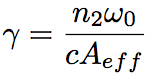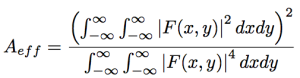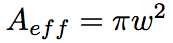Nonlinear optical effect is characterized by nonlinear coefficient, γ. Since the nonlinear coefficient, γ, is related to the nonlinear refractive index coefficient, n2, and the confinement of light in an optical fiber, it is useful for evaluating the nonlinearity. The nonlinear coefficient, γ, is given as follows.Aeff is an effective core cross-section, which represents a degree of optical confinement in an optical fiber. Provided a mode distribution in an optical fiber in the cross-sectional direction, F(x,y), Aff is expressed by the following equation.For SMF, since a mode distribution in an optical fiber is approximated by a Gaussian function, the effective core cross-section, Aeff, is simply given by the following equation.The nonlinear coefficient, γ, can be measured by using self phase modulation, cross phase modulation, four-wave mixing, optical soliton, and so on.